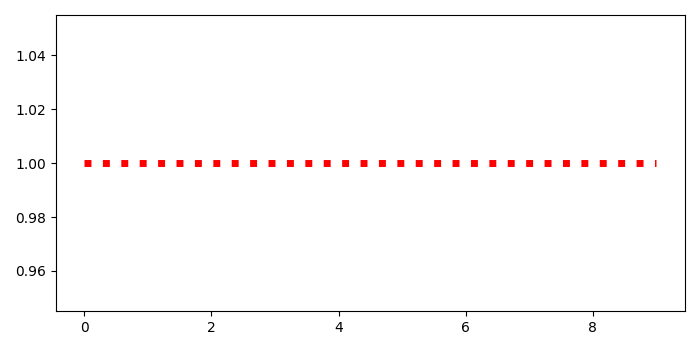# How to plot 1D data at a given Y-value with PyLab using Matplotlib?

To plot 1D data at a given Y-value with pyplot, we can take the following steps−

• Initialize y value.
• Create x and y data points using numpy. zeros_like helps to return an array of zeros with the same shape and type as a given array and add y-value for y data points.
• Plot x and y with linestyle=dotted, color=red, and linewidth=5.
• To display the figure, use show() method.

## Example

import numpy as np
from matplotlib import pyplot as plt
plt.rcParams["figure.figsize"] = [7.00, 3.50]
plt.rcParams["figure.autolayout"] = True
y_value = 1
x = np.arange(10)
y = np.zeros_like(x) + y_value
plt.plot(x, y, ls='dotted', c='red', lw=5)
plt.show()

## Output